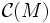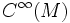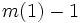# Connection algebra

Let$M$ be a differential manifold. The connection algebra of$M$, denoted$\mathcal{C}(M)$, is defined as follows. Consider the Lie algebra of first-order differential operators on$M$, and treat it as a$C^\infty(M)$-bimodule. Take the tensor algebra generated by this as a$C^\infty(M)$-bimodule, and quotient it by the two-sided ideal generated by$m(1) - 1$. Here$m(1)$ is the differential operator obtained as multiplication by the constant function$1$.
The quotient algebra we get is termed the connection algebra on$M$.